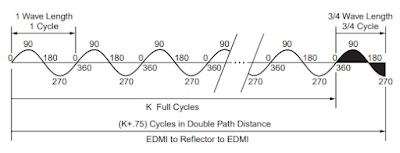# How to Measure Using EDMs in Surveying? | Electronic Distance Measurement

The distance of a survey line can be measured electronically if the velocity and travel time of electromagnetic energy propagated along the survey line is determined. The terrestrial electronic measurement instruments (EDMIs) measure the travel time, by comparing the phase of the outgoing measurement signal to the phase of the signal returning from the remote end of the line.

Hence, the phase difference is the function of the double path travel of the measurement signal. The distance is calculated from the below equation:

D = VT/2

V = The velocity of electromagnetic energy in the atmosphere

T = The double path travel time determined using the phase difference

If Δф is the phase difference observed from the fine or shortest wavelength measurement signal, then the total travel time is given by,

T = k (1/f) + Δф /2π (1/f)

Where, f = the frequency of the measurement signal, k = the integer number of full cycles in the double path distancePhase Shift Principle of Distance Measurement

The value of k is determined by measuring the phase difference of one or more coarse or long-wavelength signals to resolve the distance to the nearest full cycle of the fine wavelength.

EDMIs are classified based on the type of energy used to carry the measurement signal or by the maximum measurement range of the system. The carried energy used is visible light, infrared light, laser (red light), and microwaves.

The velocity of electromagnetic energy in the atmosphere is given by:

V = c/n

where 'c' is the velocity in a vacuum, 299,792,158 meters/second, and n is the atmospheric index of refraction for the conditions at the time of observation.